Name__________________________________ #____  St. Mary's HS Physics Snell's Law HW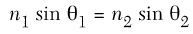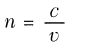1. A ray of monochromatic light (f = 5.09 x 1014 Hz) traveling in air is incident on an interface with a liquid (n = 1.4) at an angle of 45.o. a) Find the angle of refraction _____  b) Draw the refracted ray c) and the reflected ray.2. If the angle of incidence in air is 30.o, what is the angle of refraction in crown glass?

Draw the refraction diagram (be sure to also include the reflected beam)

 Air Crown Glass
 3. The diagram below shows a ray of light passing from medium X into air.  What is the absolute index of refraction of medium X?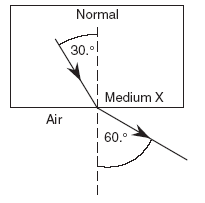(Questions 4,5) A monochromatic beam of yellow light, AB, is incident upon a Lucite block in air at an angle of 33°.  Find the angle of refraction. Draw the refracted ray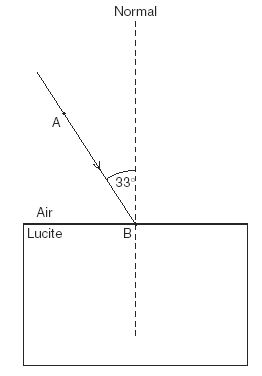Base your answers to questions 6 and 7 on the information and diagram below. In the diagram, a light ray, R, strikes the boundary of air and water.

6. Using a protractor, determine the angle of incidence. (hint: Draw the normal first)

7. Using a protractor and straightedge, find and draw the refracted ray on the diagram below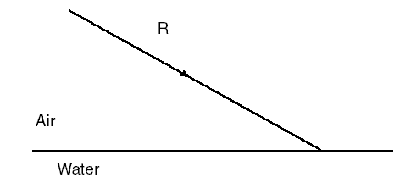(8 - 10) The diagram below which shows a ray of monochromatic light (f = 5.09 × 1014 hertz) passing through a flint glass prism.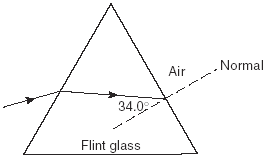8. Calculate the angle of refraction (in degrees) of the light ray as it enters the air from the flint glass prism.       9.  Using a protractor and a straightedge, construct the refracted light ray in the air on the diagram 10.  What is the speed of the light ray in flint glass? (answer in scientific notation)